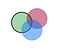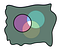# Invert polygons for fun and new functionality

Today’s story is inspired by a feature request opened for BigQuery GIS. The request, as I understand it, is to provide aggregate version of `ST_Intersect` or `ST_Intersection`. BigQuery GIS has `ST_Union_Agg`, but no `ST_Intersection_Agg`. Frankly, I still wonder about a use case when this would be needed, but let’s create such a function anyway.

The reason this is interesting is we’ll use complementary polygons here. Usually they cause troubles when a polygon with incorrect orientation is loaded to BigQuery, but here we’ll use them to our advantage.

Short refresher: on a sphere, every polygon has a complementary polygon. For example, a polygon that describes the Earth’s continents would have a complementary polygon that describes the Earth’s oceans. They have the same boundary, but all inner points of the first polygon are outer points of the second polygon, and vice versa.

How can we make a complementary polygon for a polygon? A simple solution in BigQuery is to subtract it from the whole sphere. What’s left is complementary polygon. We can define a function for this:

`create temp function ST_Invert(g GEOGRAPHY) AS  (  ST_Difference(ST_GeogFromText('fullglobe'), g)); `

Note that for polygons `ST_Invert` function follows all the logic rules of set complement, and `ST_Invert(ST_Invert(foo))` equals to `foo`. Note that this only holds true for polygon interior, this does not work for points or lines: the `ST_Invert` of any 0 or 1 dimensional shape returns full globe.

How can we use that to create common intersection?

Intersection = A ∩ B ∩ C ∩ ….

Let’s invert this equality, and use logic rules to simplify the result

¬Intersection = ¬(A ∩ B ∩ C) = (¬A) ∪ (¬B) ∪ (¬C) ∪ ….

So complement of intersection is union of complements. And we already have a function to compute union, `ST_UNION_AGG`!

Another way to arrive to this formula is graphically.Input shapes

The points that belong to intersection in the middle are points that belong to every polygon in the set. It’s complement, or points outside of the common intersection are points that are outside of at least one of the input polygon. I.e. complement of the common intersection is the union of complements:Complementary shapes

We can now define our intersection function:

`create temp function ST_Intersection_Agg(poly GEOGRAPHY) AS  (  ST_Invert(ST_Union_Agg(ST_Invert(poly)))); `

Again, a reminder that this only works correctly for polygons interiors, don’t use it with points or lines input, or when the intersection result is a point or a line. E.g. for polygons that intersect on a single point this function returns empty geometry.

Hi, I'm TL of BigQuery GIS project. Posting small recipes and various notes for BQ GIS users.

## More from Michael Entin

Hi, I'm TL of BigQuery GIS project. Posting small recipes and various notes for BQ GIS users.